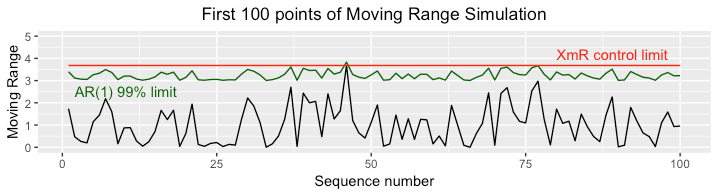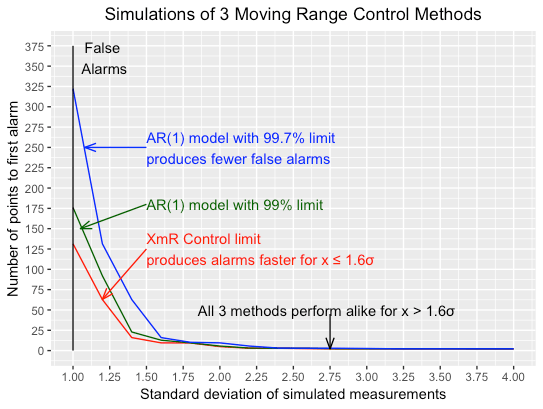# More About the Math of the Process Behavior Chart

[latexpage]

In statistics on time series with “moving” in their name, each value is correlated with past and future neighbors — that is, the series is autocorrelated. It affects the way you can use these statistics to detect anomalies and issue alarms.

The moving range in the XmR chart is a case in point. Its autocorrelation in the moving range chart is self-inflicted. It is autocorrelated by construction, regardless of whether the raw data themselves are.

Some raw data are autocorrelated. For example, when you issue a replenishment order for a part by pulling a Kanban from a bin, you are assuming that the demand for a coming period to match that of the period that just elapsed, with minor fluctuations. Implicitly, you are leveraging the autocorrelation of the part consumption across periods.

On the other hand, if a physical characteristic of a manufactured part is the sum of a constant and noise, then the noises are independent, and therefore uncorrelated. Taking moving ranges introduces an autocorrelation between consecutive values that is absent in the raw data.

## The Autocorrelation of Moving Ranges

As Don Wheeler acknowledges:

“Since each individual value is used to create two moving ranges, the computations can create correlations within the moving range values.”

He even included the following example to dramatize this point:

Wheeler points out that this prevents the use of runs in analyzing the chart. A chart is supposed to let the reader see patterns but the presence of autocorrelation here makes the most visible patterns an artefact of the technique instead of real information.

## Two Strategies to Deal With Autocorrelation

If you don’t want to ignore the autocorrelation of moving ranges, you can:

1. Replace them with a statistic that doesn’t have autocorrelation.
2. Use a model that takes it into consideration instead of the plain upper control limit of the mR chart.

### Eliminating autocorrelation by skipping every other point

So why choose a statistic that creates artificial complexity? One way to get rid of autocorrelation is to only include every other point in the range chart. If you have a series of measurements$X_1,...,X_n,...$, two consecutive values of the moving range are$R_i = \left | X_i - X_{i-1} \right |$ and$R_{i+1} = \left | X_{i+1} - X_i \right |$ are correlated because they have the term$X_i$ in common.

If you skip every other point, two consecutive points in the range chart will be$R_i = \left | X_i - X_{i-1} \right |$ and$R_{i+2} = \left | X_{i+2} - X_{i+1} \right |$ that have no common term. They are functions of different independent variables and therefore independent. If you see a run on this chart, it is as meaningful as on the X chart. The downside is that you are accumulating moving range points at half the speed of the X chart.

### Using an autoregressive model

If you want to plot every moving range, you need to use a different and slightly more complicated model, called AR(1), of the form:$R_{i+1} = \mu_R + \alpha\times \left (R_i -\mu_R \right ) + W'_{i+1}$

where$\alpha$, like$\mu_R$, are coefficients estimated on a training set of data and the$W'_i$ are independent, identically distributed noises. On future data, we can predict$R_{i+1}$ from$R_{i}$ and issue alarms when the$W'_i$ exceed a treshold.

### Visualizing the AR(1) model

It is visually less appealing that than the mR chart, because you don’t have an Upper Control Limit in the form of a flat, straight line. This is seen in the following figure, generated from a simulation with both limits corresponding to a 99% level, or p = 1%:The real issue is whether it performs any better in terms of avoiding false alarms and effectively flagging real ones. To answer this question, we need to get quantitative.

## Quantification of Moving Range Autocorrelation

If you have a series of measurements$X_1,...,X_n,...$, two consecutive values of the moving range are$R_i = \left | X_i - X_{i-1} \right |$ and$R_{i+1} = \left | X_{i+1} - X_i \right |$ have the term$X_i$ in common.

### Visualization

If, instead of the absolute values $R_i$ you take the differences$D_i = X_i - X_{i-1} = W_i - W_{i-1}$, where$W_i = X_i - \mu$ you can calculate the correlation between$D_i$ and$D_{i+1}$.

The cross terms vanish in the expected value of$D_i\times D_{i+1}$ and therefore:$E(D_i\times D_{i+1}) = -E(W_i^2) = -\sigma^2$

Since$D_i$ is the sum of two independent, centered Gaussians with standard deviation$\sigma$,$E(D_i^2) = E(D_{i+1}^2) = 2\sigma^2$
And the correlation between$D_i$ and$D_{i+1}$ is therefore -1/2.

The ranges$R_i$ are the absolute values of the$D_i$s and the math is not so easy. You can, however, estimate the correlation between$R_i$ and$R_{i+1}$ from 100,000 simulated values at 0.224. As seen in the following figures, it is too low to stand out in a scatterplot, yet has a p-value of$2.2\times10^{-16}$.

The scatterplot of moving ranges is obtained by folding all the quadrants by symmetry around the axes onto the quadrant with$D_i \geq 0$ and$D_{i+1} \geq 0$. This blurs the picture so much that the autocorrelation is no longer visually obvious.

The plotting method manages to show 100,000 points on a few square inches without producing a large blob of overlapping points. The trick is to treat it as a heat map. The area is divided into small hexagons that are colored in various shades from blue to red based on the number of points they contain.

Why 100,000 points when, in his article, Wheeler uses 150 Camshaft Bearing diameters? More generally, classical SPC bases process capability studies on sets of a few dozen points, essentially because the IT of the time it was developed did not allow you to work with larger data sets.

Today, final test at the end of a car engine assembly line produces a vector of characteristics every 30 seconds, or about 1,600 times per day. To get 100,000 actual data points, you query the history database for the past 63 work days. Simulating 100,000 points, today, is instantaneous.

### Comparative performance

The point of the moving range chart is to detect shifts in the size of the noise, which is determined by its standard deviation$\sigma$.

If we use our simulated 100,000 points with$\sigma =1$ as a training set, the ideal control system would never issue an alarm as long as$\sigma =1$ and would immediately react when it shifts to$\sigma > 1$.

No real method can actually do this. They all generate some false alarms and do not always notice a shift immediately but, using simulated testing sets, we can measure how many points it takes before a system issues a false or a real alarm.

Using 10 simulated testing sets, and values of$\sigma$ from 1 to 4, we count how many points we go through before issuing an alarm, and take the average over all the simulations. The best performance is a high number for false alarms at$\sigma =1$ and a low number for real alarms$\sigma > 1$. The following figure compares, on those terms, the following three methods:

1. The upper control limit (UCL) for the mR chart.
2. The AR(1) 99% limit for p = 1%, the same level as the UCL.
3. The AR(1) 99.7% limit for p = 0.3%, the same level as the control limits of the X chart.The chart shows that the autoregressive model produces fewer false alarms and is as effective at the UCL for$\sigma\geq 1.6$. For smaller shifts in$\sigma$, the UCL responds faster. Again, the relative importance of avoiding false alarms versus rapidly detecting small shifts in $\sigma$ depends on the maturity of the process.

## Conclusions

Manufacturing, engineering, and business in general produce all sorts of time series and all business professionals have to analyze them in some fashion.

### Plot the time series

As pointed out by authors like Don Wheeler or Mark Graban, the worst possible way to use this data is to look only at the last value. Because it’s time-dependent data, you need to consider its history by plotting values against time, which is easier to do if it is just a number, as opposed to a multidimensional vector.

### Consider the meaning of the data

Before applying any tool to a time series, you need to ponder what the plot is visually suggesting in light of the nature and origin of the data. As discussed in my commments on Mark Graban’s article on Oscar TV Viewership, it makes a difference whether the numbers represent the performance of a baseball player, a rep’s sales volume , or the torque produced by an engine.

### Use a panoply of analytical tools

Then you can apply a variety of tools to confirm or refute conjectures the plot suggests, or to identify patterns that are plausible given the nature of the data but do not stand out in the plots. Looking at an mR chart, for example, you would not guess that consecutive values are correlated and its not visible in the scatter plot of pairs of consecutive values. You assume they are correlated based on the nature of the moving range, and calculations confirm it.

### The XmR chart is not a panacea

In another paper, Wheeler identifies W.J. Jennett as the creator of the XmR chart in the UK in 1942. Wheeler then explains that he brought it back from obscurity in the 1980s and has since been promoting it as a universal tool for analyzing time series.

Wheeler’s view is far from the mainstream in time series analysis. The XmR chart is nowhere to be found in the technical literature on this topic and only has at most brief mentions in the literature on statistical quality. According to Wheeler, even Deming didn’t know about it in 1985.

### Swiss Army knives are a last resort

In this article, Wheeler also describes the XmR chart as a “Swiss Army knife.” The Swiss Army knife, however, is a tool of last resort. It has many functions but doesn’t perform any of them as well as a special purpose tool. You can use it to open a can of beans but you don’t unless you have to, because a specialized can opener works better.

As discussed in an earlier post, the only multifunction tools that outperforms special-purpose rivals is the computer, and it has changed the game in data science. Like all the tools of SPC, the XmR chart predates its invention.